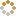﻿ N-B-U-T Online Judge ::  Modular Inverse
• ###  Modular Inverse

• 时间限制: 2000 ms　内存限制: 65535 K
• 问题描述
• The modular modular multiplicative inverse of an integer a modulo m is an integer x such that `a-1≡x (mod m)`. This is equivalent to `ax≡1 (mod m)`.
• 输入
• There are multiple test cases. The first line of input is an integer T ≈ 2000 indicating the number of test cases.

Each test case contains two integers 0 < a ≤ 1000 and 0 < m ≤ 1000.
• 输出
• For each test case, output the smallest positive x. If such x doesn't exist, output "Not Exist".
• 样例输入
• ```3
3 11
4 12
5 13```
• 样例输出
• ```4
Not Exist
8```
• 提示
• `无`
• 来源
• `浙江2012年省赛J题`
• 操作
﻿